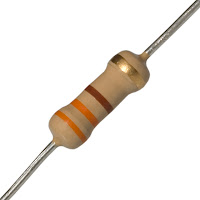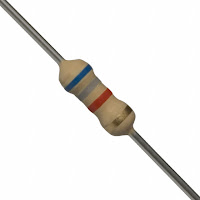### How to Find the Value of a Resistor by Color Codes

To calculate the value of a resistor using the color coded stripes on the resistor, use the following procedure.

Step One:   Turn the resistor so that the gold or silver stripe is at the right end of the resistor.

Step Two:   Look at the color of the first two stripes on the left end. These correspond to the first two digits of the resistor value. Use the table given below to determine the first two digits.

Step Three:  Look at the third stripe from the left. This corresponds to a multiplication value. Find the value using the table below.

Step Four:   Multiply the two digit number from step two by the number from step three. This is the value of the resistor n ohms. The fourth stripe indicates the accuracy of the resistor. A gold stripe means the value of the resistor may vary by 5% from the value given by the stripes.

Resistor Color Codes (with gold or silver strip on right end)Follow the above procedure with the examples below and soon you will be able to quickly determine the value of a resistor by just a glance at the color coded stripes.

Examples

Example1:
You are given a resistor whose stripes are colored from left to right as brown, black, orange, gold. Find the resistance value.Step One:  The gold stripe is on the right so go to Step Two.

Step Two:  The first stripe is brown which has a value of 1. The second stripe is black which has a value of 0. Therefore the first two digits of the resistance value are 10.

Step Three:  The third stripe is orange which means x 1,000.

Step Four:  The value of the resistance is found as 10 x 1000 = 10,000 ohms (10 kilohms = 10 kohms).

The gold stripe means the actual value of the resistor mar vary by 5% meaning the actual value will be somewhere between 9,500 ohms and 10,500 ohms. (Since 5% of 10,000 = 0.05 x 10,000 = 500)

Example2:
You are given a resistor whose stripes are colored from left to right as orange, orange, brown, silver. Find the resistance value.Step One:  The silver stripe is on the right so go to Step Two.

Step Two:  The first stripe is orange which has a value of 3. The second stripe is orange which has a value of 3. Therefore the first two digits of the resistance value are 33.

Step Three:  The third stripe is brown which means x 10.

Step Four:  The value of the resistance is found as 33 x 10 = 330 ohms.

The silver stripe means the actual value of the resistor mar vary by 10% meaning the actual value will be between 297 ohms and 363 ohms. (Since 10% of 330 = 0.10 x 330 = 33)

Example3:
You are given a resistor whose stripes are colored from left to right as blue, gray, red, gold. Find the resistance value.Step One: The gold stripe is on the right so go to Step Two.

Step Two: The first stripe is blue which has a value of 6. The second stripe is gray which has a value of 8. Therefore the first two digits of the resistance value are 68.

Step Three: The third stripe is red which means x 100.

Step Four: The value of the resistance is found as 68 x 100 = 6800 ohms (6.8 kilohms = 6.8 kohms).

The gold stripe means the actual value of the resistor mar vary by 5% meaning the actual value will be somewhere between 6,460 ohms and 7,140 ohms. (Since 5% of 6,800 = 0.05 x 6,800 = 340)

Example 4:
You are given a resistor whose stripes are colored from left to right as green, brown, black, gold. Find the resistance value.Step One: The gold stripe is on the right so go to Step Two.

Step Two: The first stripe is green which has a value of 5. The second stripe is brown which has a value of 1. Therefore the first two digits of the resistance value are 51.

Step Three: The third stripe is black which means x 1.

Step Four: The value of the resistance is found as 51 x 1 = 51 ohms.

The gold stripe means the actual value of the resistor mar vary by 5% meaning the actual value will be somewhere between 48.45 ohms and 53.55 ohms. (Since 5% of 51 = 0.05 x 51 = 2.55)

Other Resistor Information

There are some more rules that may be useful when working with resistors. You do not need to know them but if you need a resistor with a value that you do not have, you my be able to use the following information to create the value of resistor you need.

First Rule for Resistors : Series Connection

When two resistors are connected in series, as shown in Figure 1, the new resistance between points A and B is R1 + R2.

The resistors add together. For example if R1 = 500 ohms and R2 = 250 ohms then the resistance between points A and B would be R1 + R2 = 500 + 250 = 750 ohms.

Second Rule for Resistors : Parallel Connection

When two resistors are connected in parallel, as shown in Figure 2, the new resistance is smaller than either R1 or R2. The new resistance between points A and B is (R1 x R2) / (R1 + R2).

For example, if R1 = 500 and R2 = 250 then the resistance between points A and B = (500 x 250) / (500 + 250) = (125,000) / (750) = 167 ohms. If R1 = R2 then the new resistance is just R1 / 2.

Using these two rules, resistors can be combined to form new resistance values.